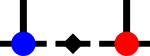SyTendense_prod_helper.h File Reference

Helper functions for the dense products. More...

#include "inc/util/limvec.h"
#include "inc/dense/dense_transpose.h"Include dependency graph for dense_prod_helper.h:This graph shows which files directly or indirectly include this file:

## Namespaces

syten
Syten namespace.

syten::DenseProduct
Contains helper functions related to the product of two dense tensors, see syten::prodD() for the main entry point.

## Functions

template<Rank frank, Rank srank>
void syten::DenseProduct::check_dense_dims (std::array< Index, frank > const &dimA, std::array< Index, srank > const &dimB, std::array< int, frank > const &c_a, std::array< int, srank > const &c_b)
Asserts that the dimensions of two tensors, as described by dimA and dimB, are compatible with hte product described in c_a and c_b. More...

template<Rank rank>
bool syten::DenseProduct::is_trivial_order_a (std::array< Index, rank > const &dims, std::array< int, rank > const &c_a)
Returns true if the tensor as specified by its dimensions and contraction specifier does not have to be transposed to be usable in a TTGT-based tensor transposition if it is the first tensor to be contracted. More...

template<Rank rank>
bool syten::DenseProduct::is_trivial_order_b (std::array< Index, rank > const &dims, std::array< int, rank > const &c_b)
Returns true if the tensor as specified by its dimensions and contraction specifier does not have to be transposed to be usable in a TTGT-based tensor transposition if it is the second tensor to be contracted. More...

template<Rank frank, Rank srank, Rank rrank>
bool syten::DenseProduct::is_trivial_order_r (std::array< Index, frank > const &dims_a, std::array< Index, srank > const &dims_b, std::array< int, frank > const &c_a, std::array< int, srank > const &c_b)
Returns true if r calculated as the contraction of tensors a and b defined by their dimensions and contraction specifiers does not have to be transposed according to those specifiers. More...

template<std::size_t rank>
std::vector< int > syten::DenseProduct::reverse_dims_to_vec (std::array< Index, rank > const &in)
Returns a vector with the entry of in in reversed order. More...

template<Rank summed, Rank frank, typename Scalar >
void syten::DenseProduct::transpose_a (DenseTensor< frank, Scalar > const &a, std::array< int, frank > const &c_a, DenseTensor< frank, Scalar > &out_a, std::array< int, frank > &out_c_a)
Transposition of a first/a tensor to trivial order. More...

template<Rank summed, Rank srank, typename Scalar >
void syten::DenseProduct::transpose_b (DenseTensor< srank, Scalar > const &b, std::array< int, srank > const &c_b, DenseTensor< srank, Scalar > &out_b, std::array< int, srank > &out_c_b, Conj do_conj=Conj::n())
Transposition of a second/b tensor to trivial order. More...

template<Rank frank, Rank srank>
void syten::DenseProduct::validateProductSpecifier ([[maybe_unused]] std::array< int, frank > const &c_a, [[maybe_unused]] std::array< int, srank > const &c_b, [[maybe_unused]] int summed=0)
Validates a pair of product specifiers. More...

## Detailed Description

Helper functions for the dense products.